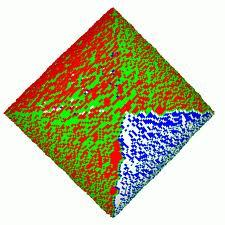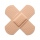# Rhombus

Find the length of the other diagonal and area of rhombus.

The perimeter of a rhombus is 40 cm and one of the diagonals is of length 10 cm.

Correct result:

d2 =  17.32 cm
S =  86.6 cm2

#### Solution:Our examples were largely sent or created by pupils and students themselves. Therefore, we would be pleased if you could send us any errors you found, spelling mistakes, or rephasing the example. Thank you!

Please write to us with your comment on the math problem or ask something. Thank you for helping each other - students, teachers, parents, and problem authors.Tips to related online calculators
Pythagorean theorem is the base for the right triangle calculator.

#### You need to know the following knowledge to solve this word math problem:

We encourage you to watch this tutorial video on this math problem:

## Next similar math problems:

• Diagonals of a rhombusOne of the diagonals of a rhombus is twice as the other. If the sum of the lengths of the diagonals is 24, find the area of the rhombus.
• Diamond diagonalsFind the diamond diagonal's lengths if the area is 156 cm2 and side is 13 cm long.
• Diagonals of a rhombus 2One diagonal of a rhombus is greater than other by 4 cm . If the area of the rhombus is 96 cm2, find the side of the rhombus.
• Diagonals of diamondFind the area and circumference of the diamond ABCD with 15m and 11m diagonals.
• Rhombus sidesfind the sides of a rhombus, it area is 550 cm square and altitude is 55cm
• A squareA square with length of diagonals 12cm give: a) Calculate the area of a square b) rhombus with the same area as the square, has one diagonal with length of 16 cm. Calculate the length of the other diagonal.
• RhombusThe rhombus has diagonal lengths of 4.2cm and 3.4cm. Calculate the length of the sides of the rhombus and its height
• Diamond diagonalsCalculate the diamonds' diagonals lengths if the diamond area is 156 cm square and the side length is 13 cm.
• Diamond diagonalsCalculate the diamond's diagonal lengths if its content is 156 cm2 and the side length is 13 cm.
• Rhombus and diagonalsThe a rhombus area is 150 cm2 and the ratio of the diagonals is 3:4. Calculate the length of its height.
• DiagonalsGiven a rhombus ABCD with a diagonalsl length of 8 cm and 12 cm. Calculate the side length and content of the rhombus.Calculate the surface of a quadrilateral prism according to the input: Area of the diamond base S1 = 2.8 m2, length of the base edge a = 14 dm, height of the prism 1,500 mm.Question No. 1: The prism has the dimensions a = 2.5 cm, b = 100 mm, c = 12 cm. What is its volume? a) 3000 cm2 b) 300 cm2 c) 3000 cm3 d) 300 cm3 Question No.2: The base of the prism is a rhombus with a side length of 30 cm and a height of 27 cm. The heigPeter taped the wound with two rectangular patches (one over the other to form the letter X). The area sealed with both patches at the same time had a content of 40cm2 and a circumference of 30cm. One of the patches was 8cm wide. What was the width of theHow long are the diagonals e, f in the diamond, if its side is 5 cm long and its area is 20 cm2?ABCD is a trapezoid that lime segment CE divided into a triangle and parallelogram as shown. Point F is the midpoint of CE, DF line passes through the center of the segment BE and the area of the triangle CDE is 3 cm2. Determine the area of the trapezoidJohn a kite, which is diamond shaped. Its diagonals are 60 cm long and 90 cm long. Calculate: a) the diamond side b) how much paper John needs to make a kite if he needs paper on both sides and needs 5% of the paper for bending.